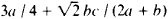# algebraic expression

## algebraic expression

[¦al·jə¦brā·ik ik′spresh·ən]
(mathematics)
An expression which is obtained by performing a finite number of the following operations on symbols representing numbers: addition, subtraction, multiplication, division, raising to a power.
McGraw-Hill Dictionary of Scientific & Technical Terms, 6E, Copyright © 2003 by The McGraw-Hill Companies, Inc.
The following article is from The Great Soviet Encyclopedia (1979). It might be outdated or ideologically biased.

## Algebraic Expression

an expression consisting of letters and numbers joined by signs denoting the operations of addition, subtraction, division, raising to whole-number powers, and extraction of roots (exponents of powers and indices of radicals must be constants). An algebraic expression is said to be rational with respect to certain literal symbols included within it if such symbols do not appear under a radical sign: for example,is rational with respect to a, b, and c. An algebraic expression is said to be integral with respect to certain literal symbols if it does not contain any division by expressions containing those symbols: for example, 3a/c + bc2 − 3ac/4 is integral with respect to a and b. If some or all of the literal symbols are considered to be variables, the algebraic expression is an algebraic function.

## algebraic expression

One or more characters or symbols associated with algebra; for example, A+B=C or A/B.
Copyright © 1981-2019 by The Computer Language Company Inc. All Rights reserved. THIS DEFINITION IS FOR PERSONAL USE ONLY. All other reproduction is strictly prohibited without permission from the publisher.
References in periodicals archive ?
Students receive immediate feedback on algorithmically generated questions with numerical or algebraic expression answers.
This can be analyzed by investigating the discriminant of the algebraic expression
The curve which expresses the relationship between substrate concentration and initial velocity has the same shape for most enzymes, a rectangular hyperbola, whose algebraic expression is given by Michaelis-Menten equation (1), which describes the relationship between initial velocity [V.sub.o] and substrate concentration, S :
Since the algebraic expression of AES algorithm is sparse and structured, it is inefficient to apply XL attacks directly.
Algebraic expression (B.9) accurately proves the correctness of statement (A.9) concerning the second order of infinitely small [delta] (infinitesimality of [delta] = O([[alpha].sup.2])) for a small inclination angle [delta], which determines the current inclination of the cross-beam ED in Figure 2.
Several researchers (see Booth, 1988; Greeno, 1991; Kieran, 1992; Lins, 1990) suggest that many of the fundamental difficulties experienced by beginning algebra students are due to their failure to identify equivalent forms of an algebraic expression. Linchevski and Livneh (1999) refer to the importance of students developing structure sense: "this means that they will be able to use equivalent structures of an expression flexibly and creatively.
At a later stage, by hanging the blue weights on the far side of the Number Balance, elements of an algebraic expression can be hidden from student view.
For example, the logic formula ([there exists][x.sub.k]) [phi] ([x.sub.i], [x.sub.p], [x.sub.k], [x.sub.i], [x.sub.m]) will be traduced by the algebraic expression [[pi].sub.-3] (R) where R is the extensions for a given Tarski's interpretation of the virtual predicate 0 and the resulting relation will have the following ordering of attributes: ([x.sub.i], [x.sub.j], [x.sub.l], [x.sub.m]).
However, as Pitso remarked, although the mnemonic constructed an empirical referent for learners, the referential relations created could not be translated into potential theoretical objects without understanding what was essential to the algebraic expression (10x--x).
And Andrew Bourke of the University of East Anglia in Norwich, England, says the new work takes "a very narrow view of inclusive fitness theory, focusing on details of algebraic expression of the theory at the expense of its essential insights.
In terms of algebraic expression the five cases that received the Campus Fraternity Excellence in Scholarship Award can noted as follows: (AW = AbC + aBc + ABc + ABC).

Site: Follow: Share:
Open / Close